# US9104510B1 - Multi-function floating point unit - Google Patents

Multi-function floating point unit Download PDF

## Info

Publication number
US9104510B1
US9104510B1 US12/772,146 US77214610A US9104510B1 US 9104510 B1 US9104510 B1 US 9104510B1 US 77214610 A US77214610 A US 77214610A US 9104510 B1 US9104510 B1 US 9104510B1
Authority
US
United States
Prior art keywords
floating point
plurality
adder
crossbar
arithmetic unit
Prior art date
Legal status (The legal status is an assumption and is not a legal conclusion. Google has not performed a legal analysis and makes no representation as to the accuracy of the status listed.)
Active, expires
Application number
US12/772,146
Inventor
Leonardo Rub
Dana Massie
Samuel Dicker
Current Assignee (The listed assignees may be inaccurate. Google has not performed a legal analysis and makes no representation or warranty as to the accuracy of the list.)
Knowles Electronics LLC
Original Assignee
Audience LLC
Priority date (The priority date is an assumption and is not a legal conclusion. Google has not performed a legal analysis and makes no representation as to the accuracy of the date listed.)
Filing date
Publication date
Priority to US22738109P priority Critical
Application filed by Audience LLC filed Critical Audience LLC
Assigned to AUDIENCE, INCORPORATED reassignment AUDIENCE, INCORPORATED ASSIGNMENT OF ASSIGNORS INTEREST (SEE DOCUMENT FOR DETAILS). Assignors: DICKER, SAMUEL, MASSIE, DANA, RUB, LEONARDO
Priority to US12/772,146 priority patent/US9104510B1/en
Publication of US9104510B1 publication Critical patent/US9104510B1/en
Application granted granted Critical
Assigned to AUDIENCE LLC reassignment AUDIENCE LLC CHANGE OF NAME (SEE DOCUMENT FOR DETAILS). Assignors: AUDIENCE, INC.
Assigned to KNOWLES ELECTRONICS, LLC reassignment KNOWLES ELECTRONICS, LLC MERGER (SEE DOCUMENT FOR DETAILS). Assignors: AUDIENCE LLC
Application status is Active legal-status Critical
Adjusted expiration legal-status Critical

## Images

•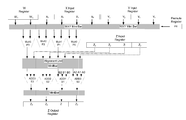•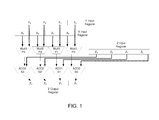•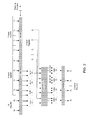•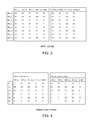•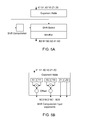•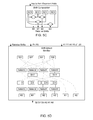••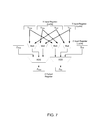•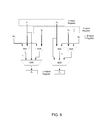•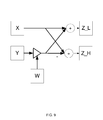•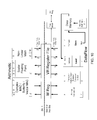•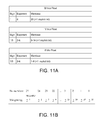•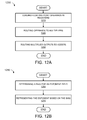## Classifications

• GPHYSICS
• G06COMPUTING; CALCULATING; COUNTING
• G06FELECTRIC DIGITAL DATA PROCESSING
• G06F7/00Methods or arrangements for processing data by operating upon the order or content of the data handled
• G06F7/38Methods or arrangements for performing computations using exclusively denominational number representation, e.g. using binary, ternary, decimal representation
• G06F7/48Methods or arrangements for performing computations using exclusively denominational number representation, e.g. using binary, ternary, decimal representation using non-contact-making devices, e.g. tube, solid state device; using unspecified devices
• G06F7/544Methods or arrangements for performing computations using exclusively denominational number representation, e.g. using binary, ternary, decimal representation using non-contact-making devices, e.g. tube, solid state device; using unspecified devices for evaluating functions by calculation
• G06F7/5443Sum of products
• GPHYSICS
• G06COMPUTING; CALCULATING; COUNTING
• G06FELECTRIC DIGITAL DATA PROCESSING
• G06F7/00Methods or arrangements for processing data by operating upon the order or content of the data handled
• G06F7/38Methods or arrangements for performing computations using exclusively denominational number representation, e.g. using binary, ternary, decimal representation
• G06F7/48Methods or arrangements for performing computations using exclusively denominational number representation, e.g. using binary, ternary, decimal representation using non-contact-making devices, e.g. tube, solid state device; using unspecified devices
• G06F7/483Computations with numbers represented by a non-linear combination of denominational numbers, e.g. rational numbers, logarithmic number system, floating-point numbers

## Abstract

Arithmetic units and methods for floating point processing are provided. In exemplary embodiments, data paths to and from multiple multipliers and adders are flexibly combined through crossbars and alignment units to allow a wide range of mathematical operations, including affine and SIMD operations. The micro-architecture for a high-performance flexible vector floating point arithmetic unit is provided, which can perform a single-cycle throughput complex multiply-and-accumulate operation, as well as a Fast Fourier Transform (radix-2 decimation-in-time) Butterfly operation.

## Description

CROSS REFERENCE TO RELATED APPLICATIONS

This application claims the benefit of U.S. Provisional Application No. 61/227,381, filed on Jul. 21, 2009, entitled “Multi-Function Floating Point Unit,” having inventors Leonardo Rub, Dana Massie, and Samuel Dicker, which is hereby incorporated by reference in its entirety.

BACKGROUND

Today, many microprocessors and digital signal processor (DSP) chips exist, such as the Intel Pentium family, the ARM microprocessors used in many portable consumer electronics devices, and Texas Instruments DSP chips such as the TI64xx family, which have multiple arithmetic functional units for performing calculations. Typically, these chips have integer arithmetic units for performing integer calculations, and floating point units (FPUs) for performing floating point format operations. Floating point is a way to represent numbers using an exponent and a mantissa and a sign bit, which offers wide dynamic range.

Floating point operation performance is limited both because traditional micro-architectures fail to support a sufficiently wide variety of operation types, and because vector operations are necessarily slowed down by required data permutations before or after the floating point operations.

SUMMARY OF THE INVENTION

In some embodiments, the present technology includes an arithmetic unit that includes a flexible vector arithmetic unit to perform a set of floating point arithmetic operations efficiently. The unit can include four or more floating point multipliers and four or more floating point adders. In some embodiments, other numbers of multipliers and adders can be implemented. The multipliers and adders are interconnected in a flexible way to allow multiple operations, including SIMD (Single instruction, multiple data) vector arithmetic, FFT (Fast Fourier Transform) Butterfly, affine operation, and dual linear interpolation. In some embodiments, the vector arithmetic unit of the present technology can be considered a complex multiply-accumulate unit (CMAC). The vector arithmetic unit of the present technology can be included in a floating point processor which executes program instructions, for example in a chip which performs digital signal processing in an audio device.

Embodiments of the present technology perform floating point operations. Multiple floating point multiplier units and multiple floating point adder units are provided. The adder units and multiplier units are interconnected to allow multiple floating point operations. The various interconnections may be implemented by full or partial crossbars. The multiple floating point operations can include an affine operation and a complex multiply-accumulate operation. The multiple floating point operations can also include a SIMD operation, dual linear interpolation operation, vector arithmetic, and FFT butterfly. The functionality of the crossbars is not limited to data permutations or re-orderings, but may include e.g. shifting operands as required to accommodate the data flow requirements for a wide range of floating point operations.

The present technology uses floating point formats to encode numbers. Often, floating point numbers are stored in 32 bits of memory per number. In order to conserve memory and/or extend the dynamic range of a floating point format, other or smaller representations of floating points may be used called “mini-floats”. These format are especially advantageous for storing numbers. One of the characteristic properties of these formats is the use of a bias in the exponent field.

BRIEF DESCRIPTION OF THE DRAWINGS

FIG. 1 illustrates an implementation of a vector arithmetic unit.

FIG. 2 illustrates an exemplary embodiment of a vector floating point arithmetic unit according to the present technology.

FIG. 3 illustrates a table of exemplary representation of a partial crossbar in a vector floating point arithmetic unit.

FIG. 4 illustrates a table of exemplary representation of a partial crossbar in a vector floating point arithmetic unit.

FIG. 5A illustrates an exemplary implementation of a partial crossbar which couples and shifts floating point multiplier outputs to create the inputs for the floating point adders according to the present technology.

FIG. 5B illustrates an exemplary implementation of exponent processing as used in the present technology.

FIG. 5C illustrates an exemplary implementation of a shift computation as used in the present technology.

FIG. 5D illustrates an exemplary implementation of a combined shift-select and partial crossbar logic as used in the present technology.

FIG. 6 illustrates an exemplary data flow within a vector floating point arithmetic unit for affine operations.

FIG. 7 illustrates an exemplary data flow within a vector floating point arithmetic unit for complex multiply-accumulate (CMAC) operations.

FIG. 8 illustrates an exemplary data flow within a vector floating point arithmetic unit for linear interpolation operations.

FIG. 9 illustrates an exemplary data flow within a vector floating point arithmetic unit for multiple (CMAC) operations to produce a butterfly operation.

FIG. 10 illustrates an exemplary micro-architecture of a floating point processor which includes a vector floating point arithmetic unit.

FIG. 11A illustrates various floating point formats as used in the present technology.

FIG. 11B illustrates the weightings of mantissa bits in a 32-bit floating point format.

FIG. 12A illustrates a flow chart of an exemplary method for computation in an arithmetic unit.

FIG. 12B illustrates a flow chart of an exemplary method for efficient representation of a floating point number with an exponent and a mantissa.

DESCRIPTION OF EXEMPLARY EMBODIMENTS

In some embodiments, the present technology includes an arithmetic unit that includes a flexible vector arithmetic unit to perform a set of floating point arithmetic operations efficiently. The unit can include multiple floating point multipliers and multiple floating point adders. An implementation of a unit may include four floating point multipliers and four multiple floating point adders. In some implementations, other numbers of multipliers and adders can be implemented. The multipliers and adders are interconnected in a flexible way to allow multiple operations, including SIMD (Single instruction, multiple data) vector arithmetic, FFT (Fast Fourier Transform) Butterfly, affine operation, and dual linear interpolation. In some embodiments, the vector arithmetic unit of the present technology can be considered a complex multiply-accumulate unit (CMAC).

The input registers X and Y can be fed into a subset of a crossbar, called a partial crossbar, or “minibar”. The input registers are connected to the WXY minibar in FIG. 2, to allow flexible routing of input operands to the multiplication units. Another minibar between the multipliers and the adders is responsible for providing the flexibility to select between different operations of the vector arithmetic unit, together with the alignment unit in FIG. 2. The output minibar allows for routing 32-bit adder output operands to whatever organization of the output Z register is required by the arithmetic operation. These three minibars are depicted in FIG. 2.

The vector arithmetic unit of the present technology combines several features. Floating point SIMD units are known in the art, as well as arithmetic units which are capable of performing some complex floating point multiply operations. There are only very few commercial processors that are capable of performing a fused floating point complex multiply and floating point accumulate, especially with the latency of two clock cycles and throughput of one cycle in the present technology at a particular clock speed. Presently available machines are not capable of performing an affine operation as well as a complex multiply accumulate, and an FFT butterfly. Additionally, the vector arithmetic unit of the present technology can perform linear interpolation operations as well.

The flexibility in the present technology arises primarily from the interconnections between the inputs and the multipliers and between the multipliers and the adders. These units are communicatively coupled with a subset of a crossbar called a minibar. A subset of a crossbar is used because a full crossbar is well known to be expensive in terms of power, wiring, and gates (i.e. silicon area), and several subsets of connections provide an ample choice of instructions, as will be explained in the present specification.

This vector arithmetic unit may include two stages: a multiplier stage containing for example four multipliers, and an addition stage containing for example four 3-way adders. Additional or fewer stages may also be implemented. In the following examples, four (32 bit) element registers are used as inputs (X register and Y register) and outputs (Z register), but the input element size could easily be other than 32 bit without loss of generality. Some of the inputs are routed to the four multipliers generating products P0, P1, P2, and P3. Other inputs are used as additive terms in the second stage, generating sums S0, S1, S2, S3. FIG. 1 is an exemplary implementation of a vector arithmetic unit. In the examples below the computations are performed in place, i.e. one of the input registers (Z) is also the output register. Two input vector registers are used, the X register and the Y register. A third register containing two element complex values (a total of 64 bits in this embodiment) called the W register can be used to improve efficiency for some operations, and is shown in this preferred embodiment. Each register may be 128-bits wide, consisting of four 32-bit sections (a.k.a. elements), labeled X3, X2, X1, X0 and Y3, Y2, Y1, Y0 respectively. Register Z may also be 128 bits wide, consisting of four 32-bit sections, labeled Z3, Z2, Z1, and Z0.

In some examples only the operations on the bottom half of the input/result vector registers is illustrated, i.e. X1, X0, Y1, Y0 and Z1, Z0. The adders that produce sums S0 and S1 can be referred to as a dual-adder, consisting of adder A for sum S0 and adder B for sum S1. The adders that produce sums S2 and S3 can be referred to as another dual-adder, consisting of another adder A to produce sum S2 and another adder B to produce sum S3.

The operation shown in FIG. 1 is Z=Z+XY. Commonly, each 32-bit section in a vector arithmetic unit as shown in FIG. 1 has sign factors sz {−1, 0, 1} such that the following operation is implemented: Zi=szi*Zi±Xi*Yi, with iε{0, 1, 2, 3}. Sign factors are not shown in FIG. 1. An additional, optional, input register shown in FIG. 2 is the so-called permutation register or permute register referred to as “PR” register. The permutation register PR typically contains 16 or fewer bits to control the minibar routing. Optional register W typically contains fewer bits than either of the X, Y, and Z registers, for example 64 bits, which form two 32-bit elements. Without using register W, the operation of an in-place vector arithmetic unit can generically be described as Z=F(Z, X, Y), wherein F is a mathematical function. With using register W, the operation can be described as Z=F(Z, W, X, Y). With using permutation register PR in addition to register W, the operation can be described as Z=F(Z, W, X, Y, PR). More specifically in FIG. 2, the operation of the vector arithmetic unit can be described separately per stage: P1=F1(X, Y, W[, PR]) and either Si=F2(P, Z) or Si=F3(P, Z, X, Y), with iε{0, 1, 2, 3}.

The present vector arithmetic unit provides several computational options in several embodiments. Assume a given register Z has elements Z0, Z1, Z2, and Z3. Assume input vectors registers X and Y are multiplied to generate partial products P0, P1, P2, and P3. Let sign factors sz0, sz1, sz2, sz3ε{−1, 0, 1} and sign factors sp0, sp1, sp2, sp3ε{−1, 1}. Note that these sign factors are not shown in FIG. 1 or FIG. 2. The vector arithmetic unit as presented includes instructions for the following computational operations by virtue of the flexibility provided through the use of partial crossbars (shown in FIG. 2):

• 1. Affine computations:
• low word output (AFF_L):
• a) Z0=sz0*Z0+sp0*P0+sp1*P1
• b) Z1=sz1*Z1+sp2*P2+sp3*P3
• or high word output (AFF_H):
• a) Z2=sz0*Z2+sp0*P0+sp1*P1
• b) Z3=sz1*Z3+sp2*P2+sp3*P3
• 2. Butterfly:
• low word additive term (BFLY_L):
• a) Z0=sz0*X0+sp0*P0+sp1*P1
• b) Z1=sz1*X1+sp2*P2+sp3*P3
• c) Z2=sz0*X0−sp0*P0−sp1*P1
• d) Z3=sz1*X1−sp2*P2−sp3*P3
• or high word additive term (BFLY_H):
• a) Z0=sz0*X2+sp0*P0+sp1*P1
• b) Z1=sz1*X3+sp2*P2+sp3*P3
• c) Z2=sz0*X2−sp0*P0−sp1*P1
• d) Z3=sz1*X3−sp2*P2−sp3*P3
• 3. SIMD:
• a) Z0=sz0*Z0+sp0*P0
• b) Z1=sz1*Z1+sp1*P1
• c) Z2=sz2*Z2+sp2*P2
• d) Z3=sz3*Z3+sp3*P3

The generation of the product terms and the specification of the sign factors helps define a particular operation. For example, if the four element input registers X and Y are considered to contain complex numbers (X0,X1) and (Y0,Y1), where the first element is real and the second element is imaginary, the following products can be produced: P0=X0*Y0, P1=X1*Y1, P2=X0*Y1, P3=X1*Y0. If in addition we define sz0=sz1=1, sp0=1, sp1=−1, sp2=1, sp3=1, the affine computation option as previously described, specifies a complex multiply-accumulate operation (a.k.a. CMAC operation). Using the previously described definitions, the specified butterfly operation is a radix-2 decimation-in-time (FFT) butterfly. To specify a SIMD operation we would also need to specify sz2 and sz3. One possible SIMD embodiment has the constraints sz0=sz1=sz2=sz3 and sp0=sp1=sp2=sp3, but another embodiment can be implemented in a more general way without any such constraints.

FIG. 3 illustrates a table representing an exemplary embodiment for the WXY minibar in FIG. 2. The input registers to the WXY minibar are X, Y, and W, and optionally the PR register. Note that registers X and Y have four elements each in this example, whereas register W only has two. The outputs of the WXY minibar are coupled with the inputs of the four multipliers. The multipliers are labeled with a number, and each multiplier has two inputs, which are labeled “a” and “b” in FIG. 3. The left-hand side of the table covers affine, butterfly and SIMD operations. The right-hand side of the table covers CMAC and linear interpretation operations.

The adders can be organized in several ways, in different embodiments. A convenient way to group the butterfly adders is into two dual adders: one for operations 2a and 2c, and the other for operations 2b and 2d. The first dual adder takes P0 and P1 as inputs, while the second one takes P2 and P3. Furthermore, the first dual adder can be used to perform 1a, or 3a and 3b. The second dual adder can be used to perform 1b, or 3c and 3d. These extensions do not modify the interface to the product terms.

Each dual adder may require part of the available data paths in the combined alignment unit and minibar as shown in FIG. 2 to perform the de-normalization needed for floating point addition. Details of exemplary implementations are in FIGS. 5A, 5B, and 5C. FIG. 5A illustrates how the exponents of operands are used to perform a shift computation, which is subsequently used to shift the mantissa of a selected operand by a number of bits based on the size of the exponents. Note that FIG. 2 contains two instances of the logic in FIG. 5A, which allows a dual affine or complex or 4-way SIMD operation to be performed in one cycle throughput. FIG. 5B illustrates more details of the exponent processing required to control the shift computation. FIG. 5C illustrates more details of the shift computation as used in the logic of FIG. 5A. For the affine and butterfly operations, a single three-way alignment (or shifting) can be performed. For the SIMD operation, 2 two-way alignments can be performed. The table in FIG. 4 illustrates how to map these operations on the hardware of FIG. 2. Alignment/shifting for any of the previously described computational options can be implemented with two shifters per dual adder, as shown in FIG. 5D, which illustrates the combined shift-select and minibar logic, as used in FIG. 5A. The term “Alignment Unit/MiniBar” in FIG. 2 denotes the same logic as the term “Shift-Select/MiniBar” in FIG. 5A, and both terms are used interchangeably throughout.

For each dual adder, the first adder is referred to as adder A, and the second adder is referred to as adder B. In FIG. 2 a dual adder generates sums S0 and S1. Denoting the corresponding dual-adder input terms A0, A1, A2, and B0, B1, B2, the table in FIG. 4 summarizes the input routing needed to support the described computational options. This routing is performed by the minibar in FIG. 5D. The appropriate routing of the adder output (using the output minibar in FIG. 2) completes the implementation of the desired operation.

FIG. 6 illustrates the data flow for the bottom (i.e. lower) half of the vector arithmetic unit outputs for an affine operation, which is characterized by the following two formulas: Z0=±Z0±(X0*Y0)±(X1*Y1), and Z1=±Z1±(X2*Y2)±(X3*Y3). As a specific example of an affine operation, FIG. 7 illustrates the data flow for a complex multiply-accumulate operation (CMAC), which is characterized by the following two formulas: Zreal=±Zreal±((Xreal*Yreal)−(Ximag*Yimag)), and Zimag=±Zimag±((Xreal*Yimag)+(Ximag*Yreal)). FIG. 8 illustrates the data flow for a linear interpolation operation, which is characterized by the following two formulas: Z0=W0*Y0+(1−W0)*X0, and Z1=W1*Y1+(1−W1)*X1. These formulas are implemented as follows: Z0=X0+W0*Y0−X0*W0, and Z1=X1+W1*Y1−X1*W1. FIG. 9 illustrates how a butterfly operation is implemented by combining two CMAC operations. The lower half of the result, Z_L, is defined as X+W*Y, whereas the upper half of the result, Z_H, is defined as X−W*Y. In this operation, any of the operands X, Y, and W may be complex.

FIG. 10 illustrates a floating point processor comprising two register files (the W register file, and the VR register file), a data memory and an arithmetic unit having a vector arithmetic unit as presented in this specification. The arithmetic unit may further comprise a scalar floating point unit and/or a non-linear mathematics unit. The floating point processor may include load logic, store logic and conversion logic, which can be configured to compress and decompress floating point operands according to various floating point formats, e.g. the formats shown in FIG. 11A.

The present technology uses floating point formats to encode numbers. Often, floating point numbers are stored in 32 bits of memory per number. In order to conserve memory and/or extend the dynamic range of a floating point format, other or smaller representations of floating points may be used called “mini-floats”. These format are especially advantageous for storing numbers. One of the characteristic properties of these formats is the use of a programmable bias in the exponent field, which increases the range of the exponent, and therefore the dynamic range of a floating point format.

A floating point number typically has a sign bit, an exponent field, and a mantissa field. A number is then represented thus
ν=(−1)Sign*2Exponent-Bias*0.{1mantissa}

Where:

s=+1 (non-negative numbers) when the sign bit is 0

s=−1 (negative numbers) when the sign bit is 1

Bias=31

Exp=Exponent+Bias

0≦Exponent+Bias≦63 (6 bit exponent field)

−31≦Exponent≦32

Mantissa=0.{1 mantissa} in binary (that is, the significand is a zero followed by the radix point followed by binary 1 concatenated with the binary bits of the mantissa). FIG. 11A illustrates various floating point formats using this representation, including uncompressed 32-bit floating points and 16-bit mini-floats. FIG. 11B illustrates the weights of the mantissa bits for the 32-bit floating point format of FIG. 11A. This floating point format provides 26 bits of precision (about 108 dB) over a dynamic range of about 385 dB, which is determined by the ratio of the maximum and minimum (smallest absolute value magnitude) number that can be represented in this format.

Due to the implicit bit, ½≦mantissa<1.0. In converting a 32-bit float to a mini-float, the decision has to be made as to how many bits of exponent and mantissa to keep, as well as what bias to use. For example, depending on the type of calculations to be performed, all floating point operands may be expected to be positive (e.g. if they signify an amount of energy or power in an audio signal). In this case no sign bit is needed for the associated mini-float format. FIG. 11A shows considerable flexibility in choosing a mini-float format (either 16 or 8 bits), wherein each choice has a particular set of minimum and maximum values, and dynamic range.

FIG. 12A illustrates a flow chart of an exemplary method 1200 for computation in an arithmetic unit. The exemplary method 1200 includes loading floating point operands in registers (step 1210); routing operands to multipliers (step 1220); and routing multiplier outputs to adders (step 1230).

• FIG. 12B illustrates a flow chart of an exemplary method 1250 for efficient representation of a floating point number with an exponent and a mantissa. The exemplary method 1250 includes determining a bias for an exponent field (step 1260) and representing the exponent based on the bias (step 1270).

Embodiments of the present technology distinguish from the prior art in that the current processing unit provides hardware support to convert standard (32 bit) floating point numbers to a completely flexible representation where the exponent bit width, bias value, and mantissa bit widths are completely determined at run time by the arguments to the instruction. Typically, hardware conversion to a mini-float format requires a limited number (1 or 2) pre-defined encoding sizes, contrary to the technology presented in this specification.

To maximize the efficient use of memory and/or bandwidth a flexible mini-floating point format is used for floating point storage. Depending on the application, the processor can store numerical data with a programmable number of exponent bits. Where the values can be negative or positive, a sign bit is also used. All remaining bits are used for the mantissa which also uses a hidden bit for all but the lowest exponent value (0), in which case the number is de-normalized. The exponent bias (offset) can also be programmed for additional flexibility, or set as a sticky bit, or alternatively programmable as a global register value which applies to a block of instructions.

In one embodiment of the present technology, the internal arithmetic format is a 32-bit float with a sign bit, a 6-bit exponent and a 26-bit mantissa including 1 hidden bit. The 32-bit float does not support de-normalized numbers, and the smallest (0) exponent and mantissa value is treated as zero. Instructions are provided to convert from the 32-bit floating point format to the 16-bit mini-float. In those conversion instructions, the number of exponent bits, the bias and whether or not a sign bit exists can be encoded in the instruction.

The primary format used for computation is the 32 bit format shown in FIG. 11A, which is also referred to as A-float or Audience-float. It has two characteristic properties in that 1) every bit pattern is a valid number representation, and 2) the inverse of every non-zero number in this format can also be represented in this format. Negative zero is treated as zero. In most cases, arithmetic operations generate only positive zeros for consistency, but negative zeros are treated as positive zeros.

Since the mantissa can be a value between ½ and 1.0 (for purposes of discussion only, other values are possible), the most significant bit mantissa bit can be a 1. This bit does not need to be stored in memory or registers, and can be discarded for storage. This bit is known as the “hidden bit” or “implicit bit” in traditional floating point nomenclature. During arithmetic operations, the internal data pipeline will restore the hidden bit to perform actual calculations, but this detail is invisible to the programmer.

 Example Values: Exponent + Value Bias Exponent Mantissa Afloat(Value) 8.0 35 4 0x1000000 24 * 0.5 0x46000000 4.0 34 3 0x1000000 23 * 0.5 0x44000000 2.0 33 2 0x1000000 22 * 0.5 0x42000000 1.0 32 1 0x1000000 21 * 0.5 0x40000000 0.5 31 0 0x1000000 20 * 0.5 0x3E000000 0.25 30 −1 0x1000000 2−1 * 0.5 0x3C000000 0.125 29 −2 0x1000000 2−2 * 0.5 0x3A000000

 Special Values can be as indicated below. Zero Exp + bias = 0, mantissa = 0 Max Value Exp + bias = 63, mantissa = all ones (0x1FFFFFF)

IEEE format uses a different scaling for the mantissa. In IEEE-754 format, for example, the mantissa is interpreted as between 1.0<mantissa<2.0.

De-normalized numbers are not supported for 32-bit floats. If exponent<0, and the mantissa=zero, then the number is interpreted as zero. The values of the mantissa in this smallest segment (where exponent=0) are still interpreted as being between ½ and 1.0 by pre-pending the hidden or implicit bit, except only for the case where the mantissa is exactly zero and the exponent is also zero.

Zero can be represented by exp+bias=0 and mantissa=0, and sign bit can be either 0 or 1. In sign magnitude systems, such as IEEE floats and A-floats, it is possible to have both a positive and a negative zero. In IEEE 754 floating point standard, zero is represented by the exponent=0. For this case, the mantissa is assumed to be zero. This is a different convention than with A-floats.

The floating point processor can implement bit-reverse ordering for the FFT, using the following guidelines for the implementation:

• 1. The computations must be done in place to save DRAM. Even in cases when the original data must be preserved the most likely scenario is that real data will be copied to a complex buffer passed to the FFT and/or a copy with format conversion will be performed.
• 2. The input and output must be in normal order. In most cases having the input in normal order and the output in bit-reverse order is adequate. However, keeping both in normal order is more user friendly.

The floating point processor implements features to make the bit-reverse step efficient, including 1) incorporate an in-place bit-reverse step with the first (radix-2) stage of the FFT without loss of efficiency, and 2) make the bit-reverse step loop over all indices needing bit-reversing rather than looping over all indices and performing an inefficient ‘if’ statement.

The implementation described herein makes use of a single vector load/store unit. The same main ideas above can be implemented with other memory interfaces such as dual load/store units.

In order to keep both input and output in normal order, bit-reverse addressing is used in one of the stages when the input and output are kept in separate buffers. With in-place computations there is no straightforward way to avoid overwriting other buffer elements. The simplest reordering is performed in a separate bit-reverse step such as:

for (ii=0; ii<N; ii++)//N: buffer size

{

• jj=bit_reverse(ii,log 2_N); //log 2_N=log 2(N),
• //number of bits in index
• if (ii<jj)
• {
• swap(buff[ii], buff[jj]);
• }
}

The reason for the ‘if’ statement above is that without it the reordering would occur twice and the buffer would end up in the original order. The problem with this separate bit-reverse step is that it may add 3N to 5N cycles to the FFT. This would be particularly detrimental for smaller values of N.

A convenient way of getting around this problem is to combine the bit-reverse step with the first stage of the FFT. Including the bit-reverse step in the first FFT stage reduces the required number of instructions significantly. However, the ‘if’ statements inside the loop are inefficient. The preferred embodiment of the vector arithmetic unit includes instructions that determine the next element index that needs bit-reversal based on a loop index or set of loop indices. These instructions do away with the need to use ‘if’ statements.

The present technology is described above with reference to exemplary embodiments. It will be apparent to those skilled in the art that various modifications may be made and other embodiments can be used without departing from the broader scope of the present technology. For example, embodiments of the present invention may be applied to any system (e.g., non speech enhancement system) utilizing AEC. Therefore, these and other variations upon the exemplary embodiments are intended to be covered by the present invention.

## Claims (19)

The invention claimed is:
1. A vector floating point arithmetic unit, comprising:
a first crossbar accommodating data flow requirements for floating point operations;
and
a second crossbar accommodating the data flow requirements for floating point operations;
wherein the first crossbar couples a plurality of input registers to a plurality of floating point multiplier units; and
wherein the second crossbar couples and aligns output products of the plurality of floating point multiplier units to a plurality of floating point adder units, the plurality of floating point adder units having a set of adder inputs and having output sums.
2. The vector floating point arithmetic unit of claim 1, wherein the floating point operations include an affine operation and a complex multiply-accumulate operation.
3. The vector floating point arithmetic unit of claim 1, wherein the floating point operations include a Fourier Transform butterfly operation and a dual linear operation.
4. The vector floating point arithmetic unit of claim 2, wherein the floating point operations further include a Fourier Transform butterfly operation and a dual linear operation.
5. The vector floating point arithmetic unit of claim 1, wherein the output products and adder inputs include mantissas and exponents.
6. The vector floating point arithmetic unit of claim 5, wherein the second crossbar further comprises shifting logic configured to right-shift the mantissa of the output product based on the size of the exponent associated with the mantissa.
7. The vector floating point arithmetic unit of claim 5, wherein the second crossbar further comprises shifting logic configured to right-shift the mantissa of an adder input by a number of bits based on a difference between the exponent of the adder input and the largest exponent from the set of adder inputs.
8. The vector floating point arithmetic unit of claim 1, wherein a radix-2 decimation-in-time Fast Fourier Transform butterfly operation is performed in one clock-cycle throughput.
9. A method for computation in an arithmetic unit, the method comprising:
routing, by a first crossbar configured to accommodate data flow requirements for floating point operations, at least part of a plurality of floating point operands to a plurality of multiplier units having output products; and
routing, by a second crossbar configured to accommodate the data flow requirements for floating point operations, the output products of the plurality of multiplier units to a plurality of adder units having a set of adder inputs and having output sums.
10. The method of claim 9, wherein the floating point operations include affine operations and a complex multiply-accumulate operation.
11. The method of claim 10, wherein the floating point operations further include a radix-2 decimation-in-time Fast Fourier Transform butterfly operation and a dual linear operation.
12. The method of claim 9, wherein the output products and the plurality of adder inputs include mantissas and exponents.
13. The method claim 12, wherein the routing by a second crossbar further comprises right-shifting the mantissa of an adder input by a number of bits based on a difference between the exponent of the adder input and the largest exponent from the set of adder inputs.
14. A floating point processor, comprising:
a register file with a read port and a write port;
a data memory coupled to the register file; and
an arithmetic unit coupled to the register file, comprising:
a scalar floating point arithmetic unit, and
a vector floating point arithmetic unit comprising:
a first crossbar accommodating data flow requirements for floating point operations, the first crossbar coupling a plurality of input registers to a plurality of floating point multiplier units having output products,
a plurality of floating point adder units having a set of adder inputs and having output sums, and
a second crossbar accommodating data flow requirements for floating point operations, the second crossbar aligning and coupling the output products of the plurality of floating point multiplier units to the plurality of floating point adder units.
15. The floating point processor of claim 14, wherein the data memory is coupled to the register file through load logic, store logic, and conversion logic.
16. The floating point processor of claim 15, wherein the load logic and the store logic are configured to operate on compressed floating point operands.
17. The floating point processor of claim 16, wherein the conversion logic is configured to compress and decompress the floating point operands.
18. The floating point processor of claim 14, wherein the output products and adder inputs include mantissas and exponents, and wherein the second crossbar further comprises shifting logic configured to right-shift the mantissa of an adder input by a number of bits based on a difference between the exponent of the adder input and the largest exponent from the set of adder inputs.
19. A vector floating point arithmetic unit, comprising:
a plurality of floating point multiplier units having output products;
a first crossbar configured to accommodate data flow requirements for floating point operations, the first crossbar coupling a plurality of input registers to the plurality of floating point multiplier units;
a plurality of floating point adder units having a set of adder inputs and having output sums; and
a second crossbar configured to accommodate data flow requirements for floating point operations, the second crossbar coupling and aligning the output products of the plurality of floating point multiplier units to the plurality of floating point adder units.
US12/772,146 2009-07-21 2010-04-30 Multi-function floating point unit Active 2032-12-13 US9104510B1 (en)

## Priority Applications (2)

Application Number Priority Date Filing Date Title
US22738109P true 2009-07-21 2009-07-21
US12/772,146 US9104510B1 (en) 2009-07-21 2010-04-30 Multi-function floating point unit

## Applications Claiming Priority (1)

Application Number Priority Date Filing Date Title
US12/772,146 US9104510B1 (en) 2009-07-21 2010-04-30 Multi-function floating point unit

## Publications (1)

Publication Number Publication Date
US9104510B1 true US9104510B1 (en) 2015-08-11

# Family

## Family Applications (1)

Application Number Title Priority Date Filing Date
US12/772,146 Active 2032-12-13 US9104510B1 (en) 2009-07-21 2010-04-30 Multi-function floating point unit

## Country Status (1)

Country Link
US (1) US9104510B1 (en)

## Cited By (2)

* Cited by examiner, † Cited by third party
Publication number Priority date Publication date Assignee Title
US20140052968A1 (en) * 2011-12-23 2014-02-20 Intel Corporation Super multiply add (super madd) instruction
US20140052969A1 (en) * 2011-12-23 2014-02-20 Intel Corporation Super multiply add (super madd) instructions with three scalar terms

## Citations (11)

* Cited by examiner, † Cited by third party
Publication number Priority date Publication date Assignee Title
US4757466A (en) * 1984-09-07 1988-07-12 Hitachi, Ltd. High speed data processing system and method
US4807183A (en) * 1985-09-27 1989-02-21 Carnegie-Mellon University Programmable interconnection chip for computer system functional modules
US5187796A (en) * 1988-03-29 1993-02-16 Computer Motion, Inc. Three-dimensional vector co-processor having I, J, and K register files and I, J, and K execution units
US5424969A (en) * 1992-02-05 1995-06-13 Fujitsu Limited Product-sum operation unit
US5657262A (en) * 1994-04-19 1997-08-12 Sgs-Thomson Microelectronics, S.A. Arithmetic and logic computation device and control method
US6240437B1 (en) * 1993-11-30 2001-05-29 Texas Instruments Incorporated Long instruction word controlling plural independent processor operations
US6480872B1 (en) * 1999-01-21 2002-11-12 Sandcraft, Inc. Floating-point and integer multiply-add and multiply-accumulate
US20040225704A1 (en) * 2003-01-31 2004-11-11 Stmicroelectronics Sa Configurable electronic circuit, in particular one dedicated to arithmetic calculations
US7225323B2 (en) * 2004-11-10 2007-05-29 Nvidia Corporation Multi-purpose floating point and integer multiply-add functional unit with multiplication-comparison test addition and exponent pipelines
US20080114826A1 (en) * 2006-10-31 2008-05-15 Eric Oliver Mejdrich Single Precision Vector Dot Product with "Word" Vector Write Mask
US20090158013A1 (en) * 2007-12-13 2009-06-18 Muff Adam J Method and Apparatus Implementing a Minimal Area Consumption Multiple Addend Floating Point Summation Function in a Vector Microprocessor

## Patent Citations (11)

* Cited by examiner, † Cited by third party
Publication number Priority date Publication date Assignee Title
US4757466A (en) * 1984-09-07 1988-07-12 Hitachi, Ltd. High speed data processing system and method
US4807183A (en) * 1985-09-27 1989-02-21 Carnegie-Mellon University Programmable interconnection chip for computer system functional modules
US5187796A (en) * 1988-03-29 1993-02-16 Computer Motion, Inc. Three-dimensional vector co-processor having I, J, and K register files and I, J, and K execution units
US5424969A (en) * 1992-02-05 1995-06-13 Fujitsu Limited Product-sum operation unit
US6240437B1 (en) * 1993-11-30 2001-05-29 Texas Instruments Incorporated Long instruction word controlling plural independent processor operations
US5657262A (en) * 1994-04-19 1997-08-12 Sgs-Thomson Microelectronics, S.A. Arithmetic and logic computation device and control method
US6480872B1 (en) * 1999-01-21 2002-11-12 Sandcraft, Inc. Floating-point and integer multiply-add and multiply-accumulate
US20040225704A1 (en) * 2003-01-31 2004-11-11 Stmicroelectronics Sa Configurable electronic circuit, in particular one dedicated to arithmetic calculations
US7225323B2 (en) * 2004-11-10 2007-05-29 Nvidia Corporation Multi-purpose floating point and integer multiply-add functional unit with multiplication-comparison test addition and exponent pipelines
US20080114826A1 (en) * 2006-10-31 2008-05-15 Eric Oliver Mejdrich Single Precision Vector Dot Product with "Word" Vector Write Mask
US20090158013A1 (en) * 2007-12-13 2009-06-18 Muff Adam J Method and Apparatus Implementing a Minimal Area Consumption Multiple Addend Floating Point Summation Function in a Vector Microprocessor

## Cited By (4)

* Cited by examiner, † Cited by third party
Publication number Priority date Publication date Assignee Title
US20140052968A1 (en) * 2011-12-23 2014-02-20 Intel Corporation Super multiply add (super madd) instruction
US20140052969A1 (en) * 2011-12-23 2014-02-20 Intel Corporation Super multiply add (super madd) instructions with three scalar terms
US9733935B2 (en) * 2011-12-23 2017-08-15 Intel Corporation Super multiply add (super madd) instruction
US9792115B2 (en) * 2011-12-23 2017-10-17 Intel Corporation Super multiply add (super MADD) instructions with three scalar terms

## Similar Documents

Publication Publication Date Title
CN103064652B (en) Correcting means controls the shift position of the data packet
US7430578B2 (en) Method and apparatus for performing multiply-add operations on packed byte data
EP2350813B1 (en) Apparatus and method for performing simd multiply-accumulate operations
US9015354B2 (en) Efficient complex multiplication and fast fourier transform (FFT) implementation on the ManArray architecture
RU2263947C2 (en) Integer-valued high order multiplication with truncation and shift in architecture with one commands flow and multiple data flows
CA2735354C (en) Programmable processor and method with wide operations
US6243732B1 (en) Data processor and data processing system
US6247036B1 (en) Processor with reconfigurable arithmetic data path
KR101851487B1 (en) Systems, apparatuses, and methods for expanding a memory source into a destination register and compressing a source register into a destination memory location
US6922716B2 (en) Method and apparatus for vector processing
US6292886B1 (en) Scalar hardware for performing SIMD operations
US20060041610A1 (en) Processor having parallel vector multiply and reduce operations with sequential semantics
US6078941A (en) Computational structure having multiple stages wherein each stage includes a pair of adders and a multiplexing circuit capable of operating in parallel
US6385632B1 (en) Fast CORDIC algorithm with sine governed termination
US7062526B1 (en) Microprocessor with rounding multiply instructions
US5631859A (en) Floating point arithmetic unit having logic for quad precision arithmetic
CN100474235C (en) Multiply-accumulate (mac) unit for single-instruction/multiple-data (simd) instructions
Swartzlander et al. FFT implementation with fused floating-point operations
US6349319B1 (en) Floating point square root and reciprocal square root computation unit in a processor
US6711602B1 (en) Data processor with flexible multiply unit
US6748521B1 (en) Microprocessor with instruction for saturating and packing data
US8024394B2 (en) Dual mode floating point multiply accumulate unit
CN103221916B (en) Multiply multiply-accumulate instruction execution
US6671797B1 (en) Microprocessor with expand instruction for forming a mask from one bit
CN103502935B (en) Vector friendly instruction format and execution

## Legal Events

Date Code Title Description
AS Assignment

Owner name: AUDIENCE, INCORPORATED, CALIFORNIA

Free format text: ASSIGNMENT OF ASSIGNORS INTEREST;ASSIGNORS:RUB, LEONARDO;MASSIE, DANA;DICKER, SAMUEL;REEL/FRAME:024321/0681

Effective date: 20100429

STCF Information on status: patent grant

Free format text: PATENTED CASE

AS Assignment

Owner name: AUDIENCE LLC, CALIFORNIA

Free format text: CHANGE OF NAME;ASSIGNOR:AUDIENCE, INC.;REEL/FRAME:037927/0424

Effective date: 20151217

Owner name: KNOWLES ELECTRONICS, LLC, ILLINOIS

Free format text: MERGER;ASSIGNOR:AUDIENCE LLC;REEL/FRAME:037927/0435

Effective date: 20151221

MAFP Maintenance fee payment

Free format text: PAYMENT OF MAINTENANCE FEE, 4TH YEAR, LARGE ENTITY (ORIGINAL EVENT CODE: M1551); ENTITY STATUS OF PATENT OWNER: LARGE ENTITY

Year of fee payment: 4